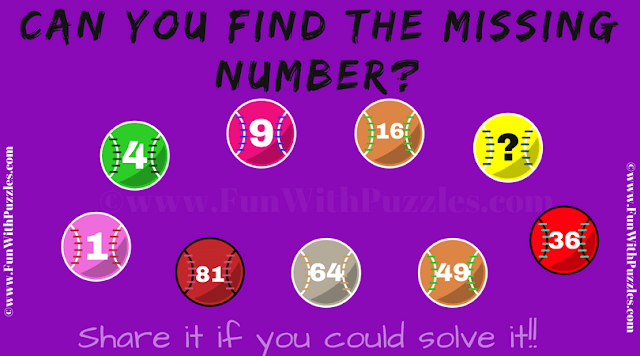This Number Balls Sequence Math Puzzle is for kids. In this Math Series Puzzle, there are 9 balls arranged in a circular fashion. Each of the ball contains a number. These numbers on the balls makes a mathematical or logical series. Your challenge is find this pattern in the series and then find the value of the missing number which will replace the question mark. So can you find the missing number in this series?Can you find the missing number?

Answer of this "Number Balls Sequence Math Puzzle ", can be viewed by clicking on button. Please do give your best try before looking at the answer.